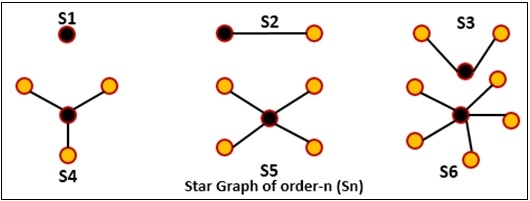# Check For Star Graph

A graph is given; we have to check the given graph is a star graph or not.

By traversing the graph, we have to find the number of vertices has degree one, and number of vertices, whose degree is n-1. (Here n is the number of vertices in the given graph). When the number of vertices with degree 1 is n-1 and a number of vertices with a degree (n-1) is one, then it is a star graph.## Input and Output

Input:
0 1 1 1
1 0 0 0
1 0 0 0
1 0 0 0

Output:
It is a star graph.

## Algorithm

checkStarGraph(graph)

Input: The given Graph.

Output: True when the graph is a star graph.

Begin
degOneVert := 0 and degNminOneGraph := 0
if graph has only one vertex, then
return true, if there is no self-loop
else if graph has two vertices, then
return true if there is only one vertex between two vertices
else
for all vertices i in the graph, do
degree := 0
for all vertices j, adjacent with i, do
degree := degree + 1
done
if degree = 1, then
degOneVert := degOneVert + 1
else if degree = n-1, then
degNminOneGraph := degNminOneGraph + 1
done

if degOneVert = n-1, and degNminOneGraph = 1, then
return true
otherwise return false
End

## Example

#include<iostream>
#define NODE 4
using namespace std;

int graph[NODE][NODE] = {
{0, 1, 1, 1},
{1, 0, 0, 0},
{1, 0, 0, 0},
{1, 0, 0, 0}
};

bool checkStarGraph() {
int degOneVert = 0, degVert = 0;    //initially vertex with degree 1 and with degree n - 1 are 0
if (NODE == 1)    //when there is only one node
return (graph == 0);

if (NODE == 2)
return (graph == 0 && graph == 1 && graph == 1 && graph == 0 );

for (int i = 0; i < NODE; i++) {    //for graph more than 2
int degree = 0;
for (int j = 0; j < NODE; j++)    //count degree for vertex i
if (graph[i][j])
degree++;
if (degree == 1)
degOneVert++;
else if (degree == NODE-1)
degVert++;
}
//when only vertex of degree n-1, and all other vertex of degree 1, it is a star graph
return (degOneVert == (NODE-1) && degVert == 1);
}

int main() {
if(checkStarGraph())
cout << "It is a star graph.";
else
cout << "It is not a star graph.";
}

## Output

It is a star graph.